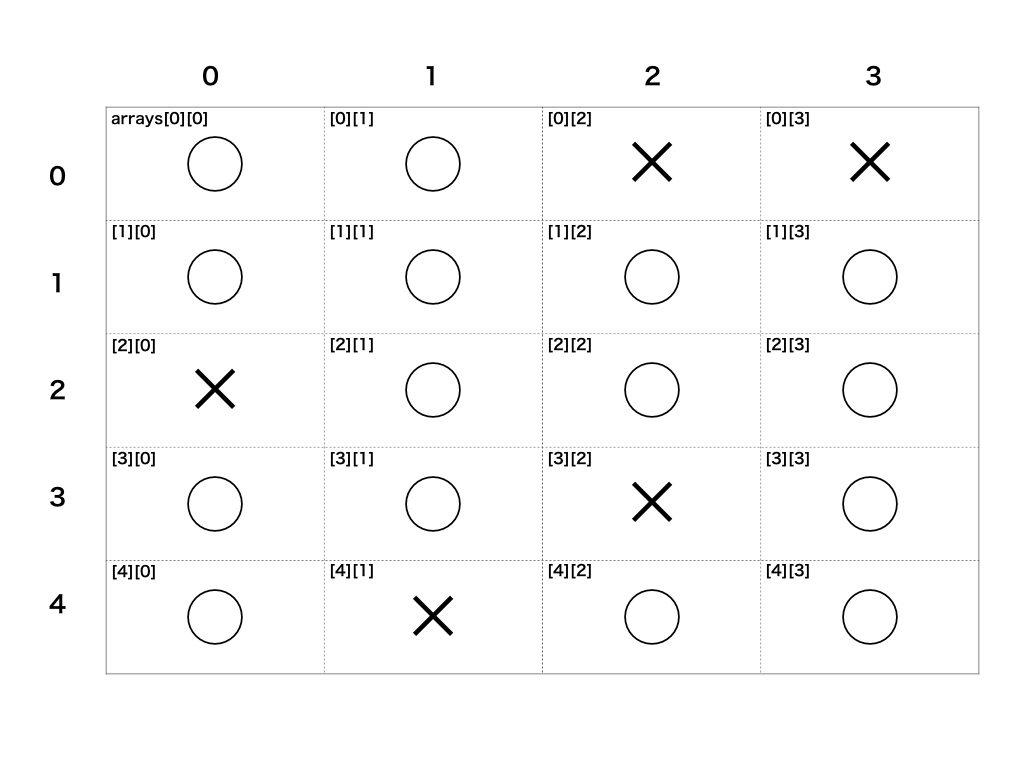# What is a two-dimensional array?

A two-dimensional array is an array that has an array as an element.

``````# Example:
number_arrays = [[1, 2, 3], [4, 5, 6], [7, 8, 9]]
color_arrays = [["red", "yellow", "blue"], ["white", "black", "gray"]]
``````

By using a two-dimensional array, two-dimensional (plane) data can be represented. Example: Representing the state of cells (◯ or ✕) with a two-dimensional array``````# ◯: 1, ✕: 0
arrays = [
[1, 1, 0, 0],
[1, 1, 1, 1],
[0, 1, 1, 1],
[1, 1, 0, 1],
[1, 0, 1, 1]
]
``````

# How to use 2D array

## Creating a two-dimensional array

A two-dimensional array can be created in the same way as a normal array.

``````# Directly define and create
arrays = [[1, 2, 3], [4, 5, 6], [7, 8, 9]]

# Define an empty array arrays and create an array in it
arrays = []
arrays << [1, 2, 3]
arrays << [4, 5, 6]
arrays << [7, 8, 9]

arrays #=> [[1, 2, 3], [4, 5, 6], [7, 8, 9]]
``````

## Get value

To get an array in a two-dimensional array Variable name [Index number of array to be acquired] Write as.

``````arrays = [[1, 2, 3], [4, 5, 6], [7, 8, 9]]

arrays #=> [1, 2, 3]
arrays #=> [4, 5, 6]
arrays #=> [7, 8, 9]
``````

Also, to get each element of the array in a two-dimensional array Variable name [Index number of array to be acquired] [Index number of element to be acquired] Write as.

``````arrays = [[1, 2, 3], [4, 5, 6], [7, 8, 9]]

arrays #=> 1
arrays #=> 2
arrays #=> 3
arrays #=> 4
arrays #=> 5
arrays #=> 6
arrays #=> 7
arrays #=> 8
arrays #=> 9
``````

# Method for 2D array

There are also methods for two-dimensional arrays.

## transpose

Using the transpose method, you can create a two-dimensional array with the rows and columns of the two-dimensional array swapped.

``````# Example:

arrays = [[1, 2, 3], [4, 5, 6], [7, 8, 9]]

If you express # arrays in a plane, it becomes as follows
# arrays = [
#   [one two Three],
# [4, 5, 6],
# [7, 8, 9]
#]

transposed_arrays = arrays.transpose
transposed_arrays #=> [[1, 4, 7], [2, 5, 8], [3, 6, 9]]

If #transposed_arrays is expressed as a plane, it will be as follows.
# transposed_arrays = [
# [1, 4, 7],
# [2, 5, 8],
# [3, 6, 9]
#]
``````

## flatten

The flatten method can be used to create a flattened two-dimensional array (the inner array is scattered).

``````# Example:

arrays = [[1, 2, 3], [4, 5, 6], [7, 8, 9]]
flattened_arrays = arrays.flatten
flattened_arrays #=> [1, 2, 3, 4, 5, 6, 7, 8, 9]
``````

# Summary

• A two-dimensional array is an array that has an array as an element.
• 2D array can be used to represent plane data
• Arrays and values in a two-dimensional array can be obtained by specifying the index number.
• There are also methods that can be used for two-dimensional arrays such as transpose and flatten

Tags:

Updated: